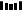### 题目描述

输入：["@.a.#","###.#","b.A.B"]输出：8

输入：["@..aA","..B#.","....b"]输出：6

1 <= grid.length <= 301 <= grid.length <= 30grid[i][j] 只含有 '.', '#', '@', 'a'-'f' 以及 'A'-'F'钥匙的数目范围是 [1, 6]，每个钥匙都对应一个不同的字母，正好打开一个对应的锁。

### 题目解析

. . . . . . . . . . B. . . . # . . . . . .. @ . A b # . . . . a. . . . # . . . . . .

### 实现代码

private class Pair {    int x, y, steps, keys;    Pair(int x, int y, int steps, int keys) {        this.x = x;        this.y = y;        this.steps = steps;        this.keys = keys;    }    @Override    public boolean equals(Object obj) {        Pair o = (Pair)obj;        if (this == o) {            return true;        }        return (this.x == o.x && this.y == o.y && this.keys == o.keys);    }    @Override    public int hashCode () {        return x * 100 + y * 10 + keys;    }}private int[] dirX = {0, 0, -1, 1};private int[] dirY = {-1, 1, 0, 0};public int shortestPathAllKeys(String[] grid) {    if (grid == null || grid.length == 0 || grid.equals("")) {        return -1;    }    int m = grid.length, n = grid.length();    Queue<Pair> queue = new LinkedList<>();    Set<Pair> visited = new HashSet<>();    int totalKeysNum = 0;    for (int i = 0; i < m; ++i) {        for (int j = 0; j < n; ++j) {            char cur = grid[i].charAt(j);            if (cur >= 'a' && cur <= 'f') {                totalKeysNum++;            }            if (cur == '@') {                Pair startPoint = new Pair(i, j, 0, 0);                queue.offer(startPoint);                visited.add(startPoint);            }        }    }    while (!queue.isEmpty()) {        Pair cur = queue.poll();        if (cur.keys == (1 << totalKeysNum) - 1) {            return cur.steps;        }        for (int i = 0; i < 4; ++i) {            int nextX = cur.x + dirX[i];            int nextY = cur.y + dirY[i];            if (nextX < 0 || nextY < 0 || nextX >= m || nextY >= n) {                continue;            }            char c = grid[nextX].charAt(nextY);            Pair nextInfo = new Pair(nextX, nextY, cur.steps + 1, cur.keys);            if (c == '#' || (c >= 'A' && c <= 'F' && ((cur.keys >> c - 'A') & 1) == 0)) {                continue;            }            if (visited.contains(nextInfo)) {                continue;            }            if (c >= 'a' && c <= 'f') {                nextInfo.keys |= (1 << c - 'a');            }            queue.offer(nextInfo);            visited.add(nextInfo);        }    }    return -1;}1.程序员

2.【GitHub

3.【算法动画：七分钟理解什么是KMP算法

4.【数据结构十大经典排序算法动画与解析，看我就够了！